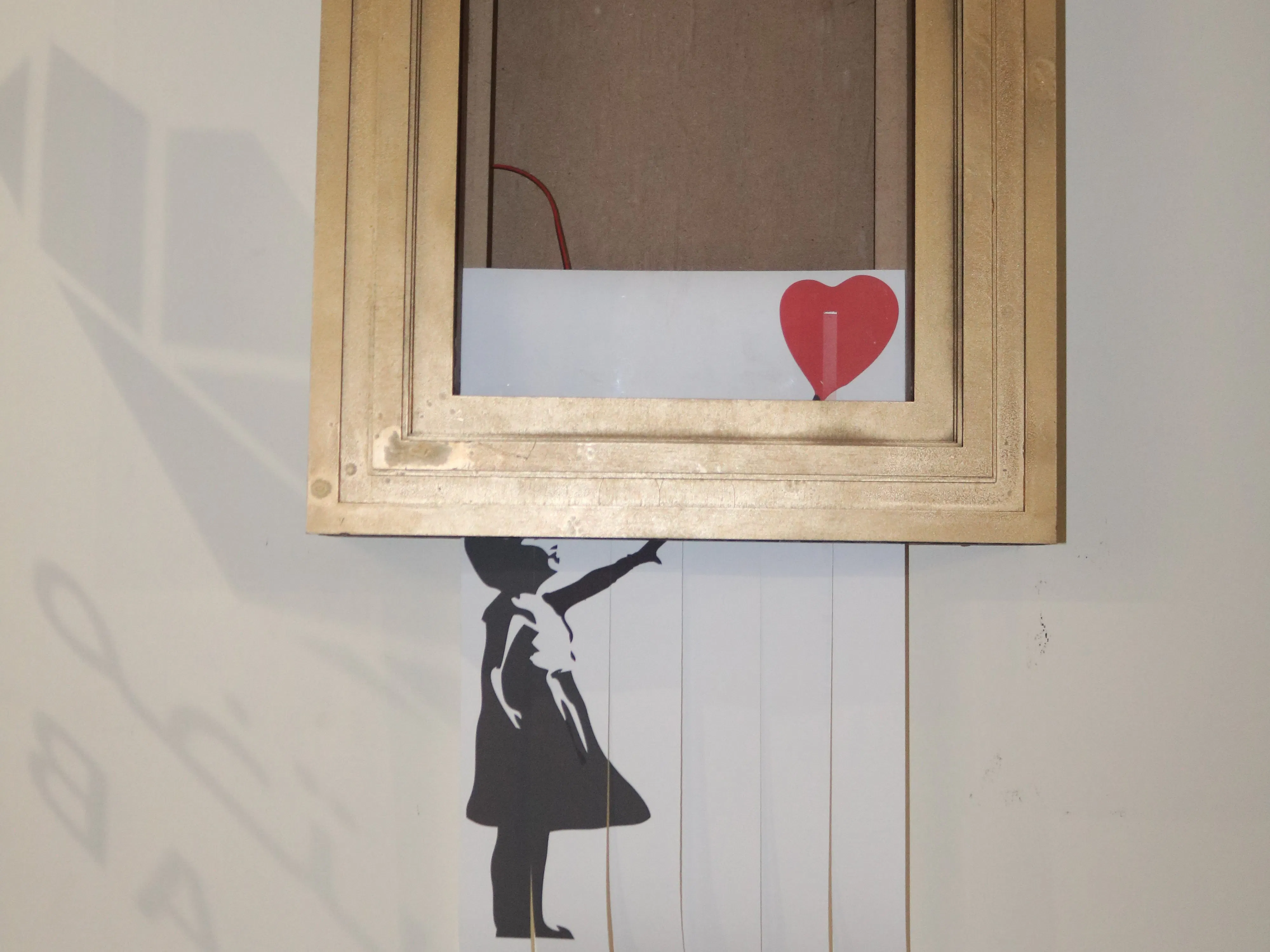# Build Your Own Banksy's "Self-Destruct Artwork Frame"

Build your own version of Banksy's "Self-Destruct Artwork Frame."

BeginnerFull instructions provided5 hours3,986## Things used in this project

### Hardware components

 DC motor (generic)
×1
 Bluetooth Low Energy (BLE) Module (Generic)
×1Arduino UNO
×1

## Code

### Banksy Arduino Code

Arduino
``` #include <SoftwareSerial.h>
SoftwareSerial BTserial(8, 9); // RX | TX
const long baudRate = 9600;
char c='3';

char x ='1';
char o = '2';
char up = '3';
char down= '4';

boolean NL = true;

int E =5;
int A = 6;
int B = 7;

int takeP=255;

void setup() {
// put your setup code here, to run once:
pinMode(E,OUTPUT);
pinMode(A,OUTPUT);
pinMode(B,OUTPUT);

Serial.begin(9600);
Serial.print("Sketch:   ");   Serial.println(__FILE__);
Serial.println(" ");

BTserial.begin(baudRate);
Serial.print("BTserial started at "); Serial.println(baudRate);
Serial.println(" ");

}

void loop() {

c = '0';
// Read from the Bluetooth module and send to the Arduino Serial Monitor
if (BTserial.available())
{
Serial.write(c);
}
if(c==x){
takepaper();
}
else if ( c== o){
shred();
}
else if (c==up){
rejectpaper();
}

if (c==10) { NL = true; }
}

void takepaper(){
digitalWrite(E,1);
analogWrite(B,155);
digitalWrite(A,0);
delay(350);
analogWrite(A,takeP);
digitalWrite(B,0);
delay(330);
analogWrite(B,150);
digitalWrite(A,0);
delay(300);
digitalWrite(A,0);
digitalWrite(B,0);

}
void shred(){
digitalWrite(E,1);
analogWrite(A,takeP);
digitalWrite(B,0);
delay(30);
analogWrite(B,takeP);
digitalWrite(A,0);
delay(3500);
analogWrite(B,0);
digitalWrite(A,0);

}
void rejectpaper(){
digitalWrite(E,1);
analogWrite(A,takeP);
digitalWrite(B,0);
delay(5000);
analogWrite(B,0);
digitalWrite(A,0);
delay(40);

}
```